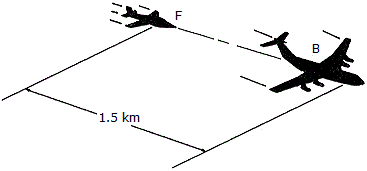Engineering Mechanics - Kinematics of Particle (KOP) - Discussion

Discussion :: Kinematics of Particle (KOP) - General Questions (Q.No.9)

9.The pilot of flighter plane F is following 1.5 km behind the pilot of bomber B. Both planes are originally traveling at 120 m/s. In an effort to pass the bomber, the pilot in F gives his plane a constant acceleration of 12 m/s2. Determine the speed at which the pilot in the bomber sees the pilot of the fighter plane pass at the start of the passing operation the bomber is decelerating at 3 m/s2. Neglect the effect of any turning.

 [A]. vF/B = 150 m/s [B]. vF/B = 367 m/s [C]. vF/B = 90 m/s [D]. vF/B = 212 m/s

Explanation:

No answer description available for this question.

 Gaurav said: (Aug 22, 2011) Solution : Here, Uf = Ub = 120 m/s (initial velocity) Relative initial velocity = Urel = Uf - Ub = 120 - 120 = 0 m/s Now, Relative acceleration(at the start of passing), Arel = 12 - (-3) = 15 m/s2 Now, Vrel^2 = Urel^2 + 2*Arel*s where, S = Distance between the aeroplanes = 1500 m We have, Vrel^2 = 0^2 + 2*15*1500 = 45000 m2/s2 Vrel = (4500)^(0.5) = 212 m/s (Answer)